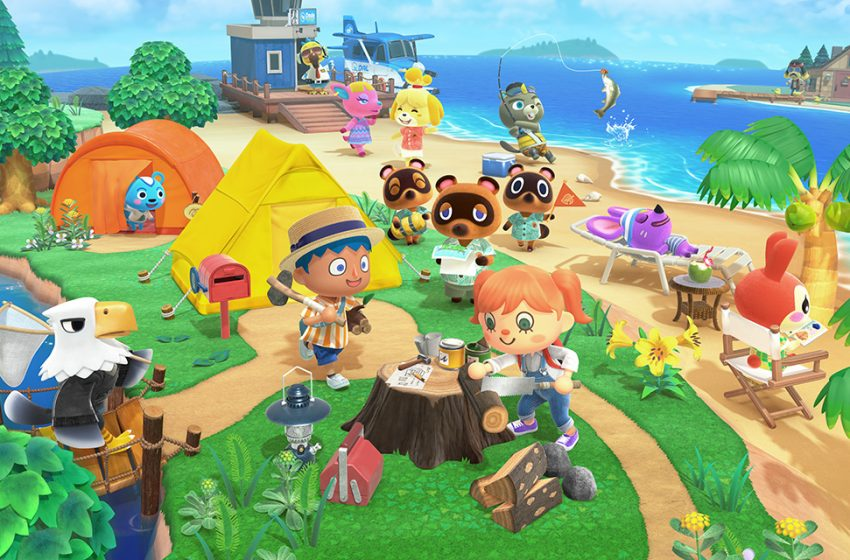# All K.K. Slider songs in Animal Crossing: New Horizons

Major chords.K.K Slider returns to Animal Crossing: New Horizons, with plenty of songs in tow. You can buy lots of his songs from the Nook Stop in Resident Services, but some you can only get by asking the man himself. The Nook Stop will feature one random song each day, and if you purchase it, then it will arrive the following day in the mail.

The below songs can only be gotten from a live K.K. Slider performance:

• Animal City
• Drivin
• Farewell

If you are wondering what to do with K.K Slider songs, you can play them on any record player, or other music playing item, except for the portable radio, which will only play random songs. You can also hang them on walls as some nice art.

The first 12 songs listed below are new songs introduced in the 2.0.0 update, with the songs after that being the original songs in the game.

## All K.K. Slider songs in New Horizons

• Chillwave
• K.K. Bashment
• K.K. Break
• K.K. Chorinho
• K.K. Dub
• K.K. Fuge
• K.K. Hip Hop
• K.K. Lovers
• K.K. Polka
• K.K. Slack-key
• Unknown Song 1
• Unknown Song 2
• Agent K.K.
• Aloha K.K.
• Animal City
• Bubblegum K.K.
• Café K.K.
• DJ K.K.
• Drivin’
• Farewell
• Forest Life
• Go K.K. Rider!
• Hypno K.K.
• I Love You
• Imperial K.K.
• K.K. Aria
• K.K. Bazaar
• K.K. Birthday – you get this as a present on your birthday
• K.K. Blues
• K.K. Bossa
• K.K. Calypso
• K.K. Casbah
• K.K. Chorale
• K.K. Condor
• K.K. Country
• K.K. Cruisin’
• K.K. D & B
• K.K. Dirge
• K.K. Disco
• K.K. Dixie
• K.K. Faire
• K.K. Flamenco
• K.K. Folk
• K.K. Fusion
• K.K. Groove
• K.K. Gumbo
• K.K. House
• K.K. Island
• K.K. Jazz
• K.K. Jongara
• K.K. Lament
• K.K. Love Song
• K.K. Lullaby
• K.K. Mambo
• K.K. Marathon
• K.K. March
• K.K. Mariachi
• K.K. Metal
• K.K. Milonga
• K.K. Moody
• K.K. Oasis
• K.K. Ragtime
• K.K. Rally
• K.K. Reggae
• K.K. Rock
• K.K. Rockabilly
• K.K. Safari
• K.K. Salsa
• K.K. Samba
• K.K. Ska
• K.K. Sonata
• K.K. Song
• K.K. Soul
• K.K. Steppe
• K.K. Stroll
• K.K. Swing
• K.K. Synth
• K.K. Tango
• K.K. Technopop
• K.K. Waltz
• K.K. Western
• K.K. Étude
• King K.K.
• Lucky K.K.
• Marine Song 2001
• Mountain Song
• Mr. K.K.
• My Place
• Neapolitan
• Only Me
• Pondering
• Rockin’ K.K.
• Soulful K.K.
• Space K.K.
• Spring Blossoms
• Stale Cupcakes
• Steep Hill
• Surfin’ K.K.
• The K. Funk
• To the Edge
• Two Days Ago
• Wandering
• Welcome Horizons – you get this the first time K.K. visits your island
• Wild World